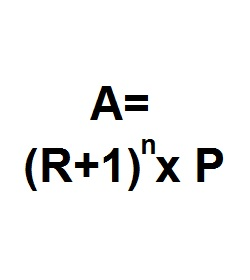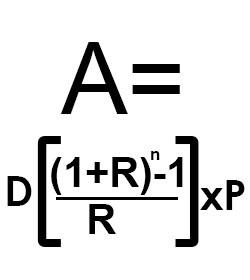Formula - Accumulated Value | Propertylogy
Don't Miss

# Formula – Accumulated Value

By on August 24, 2018A = (R+1)n x P

Where

A = Accumulated amount consisting of principal plus interest

R = Period interest rate

P = Principal

n = Number of periods

The accumulated value is an equation used to compute the interest on a single deposit.

This formula is also frequently used in the insurance industry to work out total value of whole life policies.

When there is a need to calculate accumulated value per period, it gets a little more complicated.

This can happen when there are many deposits made. And there is a requirement to work out the numbers.Where

A = Accumulated amount consisting of principal plus interest

D = Period deposit amount

R = Period interest rate

P = Principal

n = Number of periods

You May Also Like...##### How to burn more calories in the office

Send this to a friend